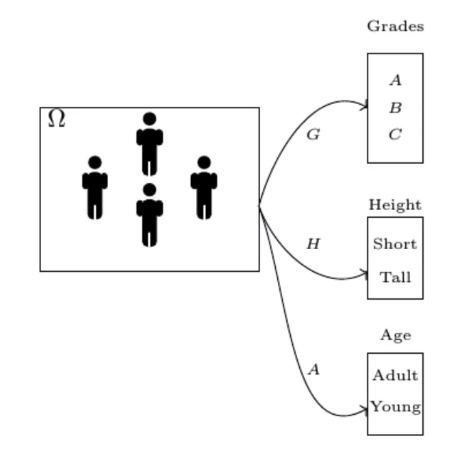# Basics: Probability Theory

Source: Deep Learning on Medium# Basics: Probability Theory

This article covers the content discussed in the Probability Theory module of the Deep Learning course and all the images are taken from the same module.

## Introduction

The probability of any event A is always ≥ 0 and it will always be ≤ 1. So, probability values lie between 0 and 1 and that’s the intuition behind using the output of Sigmoid Neuron as the probability value.

And if we have ’n’ disjoint events, the sum of the probability of the union of those events is equal to the sum of the probability of individual events.